中汽协数据显示，2018年我国乘用车销量为2370.98万辆，同比下降4.08%。其中，自主品牌下滑趋势明显，同比下滑8%。即便是这样不乐观的背景下，市场仍遵循强者恒强的规律，哈弗在年底宣告迈入500万辆俱乐部，旗下实力干将哈弗H2亦在2018年取得105363辆的销量。尤其在创享版上市后，销量一路飙升，成为车市寒冬下一股“暖流”，原因何在？不妨让我们一探究竟。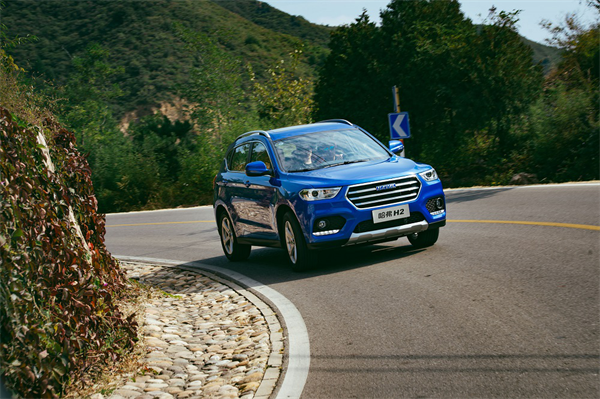颜值是第一生产力，深受年轻族群喜爱

当90后逐渐成为汽车消费主力，他们对爱车颜值的要求可谓“眼光越来越高”。哈弗H2创享版深谙其道，不仅沿用六边形和大U两种进气格栅造型，各具特色，还采用高闪烁度金属漆及高彩度珠光漆的车身，有汉密尔顿白、马尔斯红等6种颜色可选，成为小型SUV市场中名副其实的颜值担当。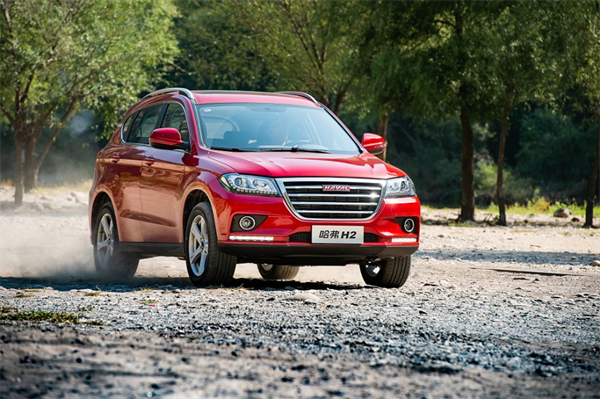此外，哈弗H2创享版还厚道地标配同级罕有的18寸铝合金轮毂，在视觉上更动感、大气，也更为实用。顶配车型还配备照射距离更远、亮度更强的LED大灯，使夜间行车变得更便捷、安全。

内饰豪华质感十足，配置丰富实用

高颜值让年轻人们对哈弗H2创享版一见钟情，而良好的口碑则源于内饰品质感及配置丰富和好用程度。在这方面哈弗H2创享版同样以高姿态引领同级市场：内饰配色加入红黑运动配色，彰显时尚气息；大面积软质材料的填充也大大提升整车质感，能给用户带来舒适豪华用车体验。在人机交互方面，新增语音控制系统，可减少驾驶者分心，保障行车安全。而主驾座椅支持6向电动调节，多功能真皮方向盘4向调节，并集成音响控制、定速巡航等17个功能按键，为驾驶更添便捷。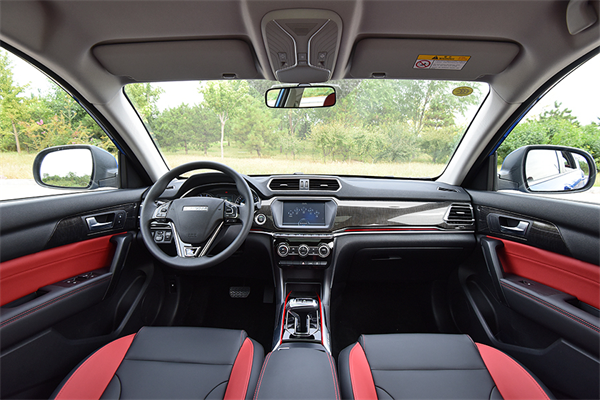另外，车内8英寸TFT触摸显示屏、支持Android及ios双系统的手机互联系统（Carlife+Carplay），能轻松连接手机，随时应用高德导航、QQ音乐、百度地图、蓝牙电话等，这一同级少有的配置同样是哈弗H2创享版吸睛的利器之一。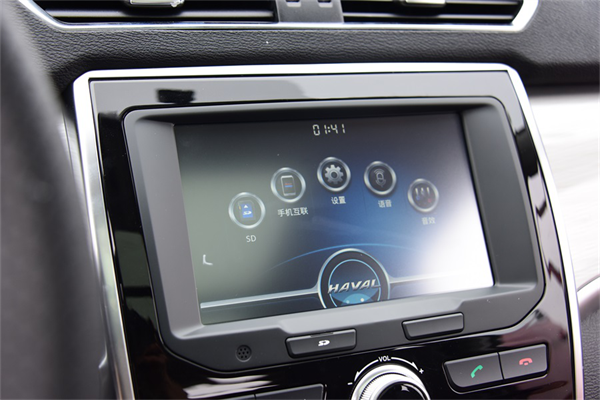同级中少有的电子档把采用“游艇式”设计，豪华感十足，换挡力度适中并支持一键回P档，设计潮流，握感舒适，使操作更便捷省力。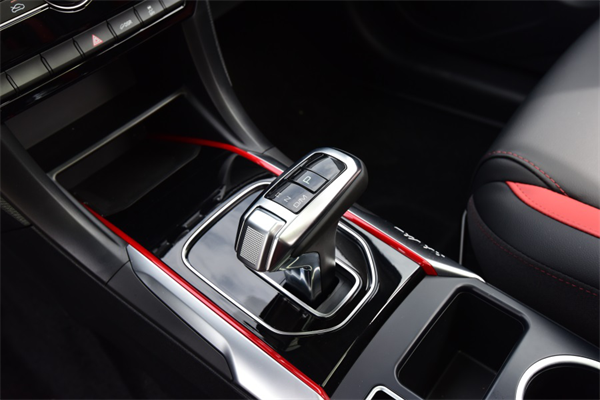配备的伟巴斯特智能全景天窗，具有锁车自动关闭、高速自动关闭及防夹等智能化功能，并拥有手动和自动两种操作模式，可与遮阳帘联动控制，人性化十足。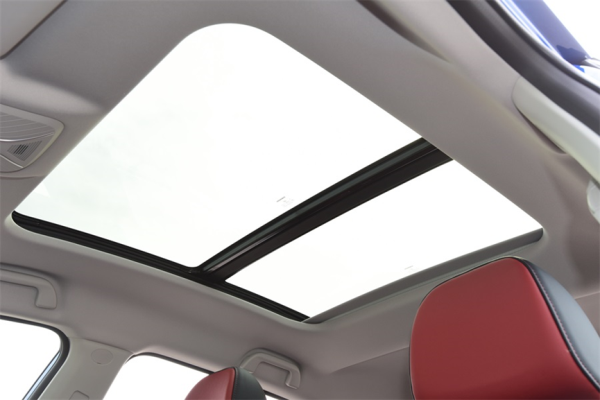澎湃动力极致体验，越级悬架脱颖而出

在当下诸多产品开始大规模采用颇具争议的三缸发动机时，哈弗H2创享版依然采用1.5T涡轮增压四缸发动机，最大功率达110kW，最大扭矩210 N·m，在动力输出、平顺性以及可靠性方面都很出色。与之搭配的是同级罕有的全新格特拉克7速湿式双离合变速箱，并有标准模式、运动模式、经济模式三种驾驶模式可选，不仅使换挡更加快速平顺，且可以做到随心切换，使驾驶变得更有乐趣。同时，结合智能启停系统，哈弗H2创享版百公里综合油耗仅为6.4L。可以说，哈弗H2创享版真正做到技术成熟，轻松驾乘，节油高效。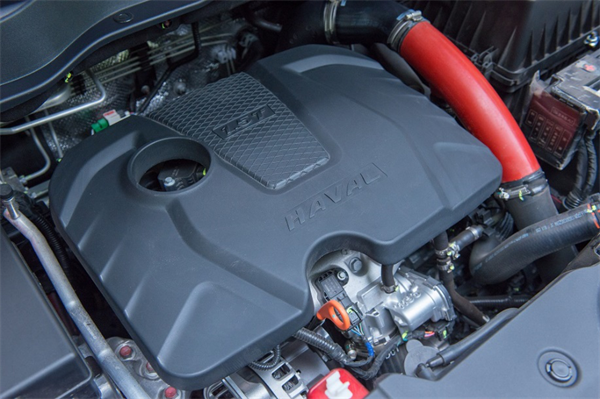作为一款“真SUV”，哈弗H2创享版在越野能力及通过性方面，也有出色表现，其搭载同级中独有的前麦弗逊、后多连杆式四轮独立悬架，具有良好的操控稳定性及行驶平顺性；22度的接近角及27度的离去角，加上165mm的离地间隙，赋予哈弗H2创享版出色的通过性；同级少有的235/55 R18轮胎，能提供更好抓地力的同时，有效保证舒适性；配备的四轮盘式制动器，采用前通风盘设计，散热性更好，刹车效能高，可以有效确保行车安全。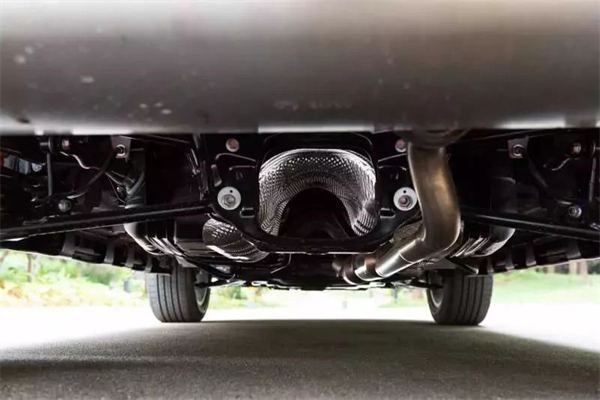安全配置丰富实用，产品过硬赢人心

从啤酒加枸杞，可乐放党参来看现如今越来越多的年轻人开始”惜命“。而在安全方面拥有C-NCAP、A-NCAP双五星评价背书的哈弗H2创享版，在主被动安全方面，同样有领先同级的表现，时刻呵护驾乘人的用车安全。像EPB智能电子手刹、AVH自动驻车功能的应用，在方便驾驶的同时，也大大提升了行车安全。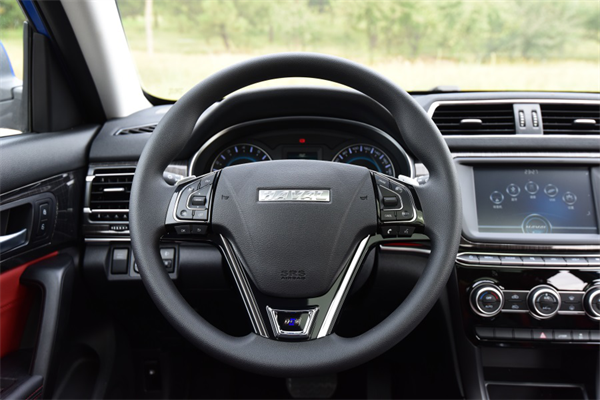其他安全配置方面，除车身使用超65%高强度钢材、6个安全气囊之外，新车还全系标配博世第九代ESP，集成了ABS制动防抱死系统、EBD电子制动力分配、TCS牵引力控制、HHC上坡辅助、RMI防侧翻系统和HDC陡坡缓降等安全配置，为驾驶安全提供了全方位的有力保障。

写在最后：通过文章可以看出哈弗H2创享版的“魔力“所在，除自身合理的产品定位及强大的产品力外，也得益于哈弗品牌对产品研发的坚持投入，以及对产品品质的“过度”追求，才造就哈弗H2自2014年诞生以来，销售总量超过70万辆，达到累积40多个月销量过万的奇迹。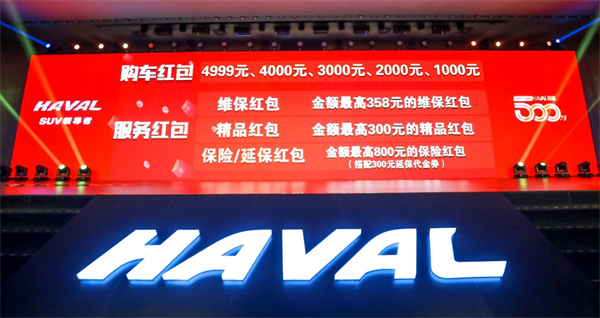值得一提的是，春节期间，哈弗还发起“庆哈弗全球销量500万，5亿红包感恩回馈”活动。在2月28日之前，不论新老哈弗车主，通过扫描活动二维码或下载“哈弗智家”APP即可参与抢红包活动分享5亿惊喜。

其中哈弗H2创享版购车红包最高可达1000元，分得红包即可直接抵扣购车款。再加上原有的“首付6万，24期0息；1年0息0月供，首付50%，尾款50%以及0首付购车”等丰富购车金融政策，以及最高3000元/台的置换政策，让打算在春节期间入手新车的小伙伴，省钱又省心。

同时，老车主们还可以享受包括维保红包、精品红包、保险红包、延保红包在内的服务红包。所有人通过扫描活动二维码或下载“哈弗智家”APP即可参与抢红包活动，享受哈弗打造的新春红包盛宴。##### 相关资讯

|分享

•已阅！握爪
•我手滑为你点赞
•128个赞！
•不明觉厉
•阅后既醉
•有钱！任性
•照片太美，我不敢看
•干货！杠杠的
•高大上
•膜拜中！
•请接受我的膝盖
•猫哥/猫妹么么哒
•神吐槽
•我只笑笑不说话
•我想静静
•窒息
##### 资讯相关车系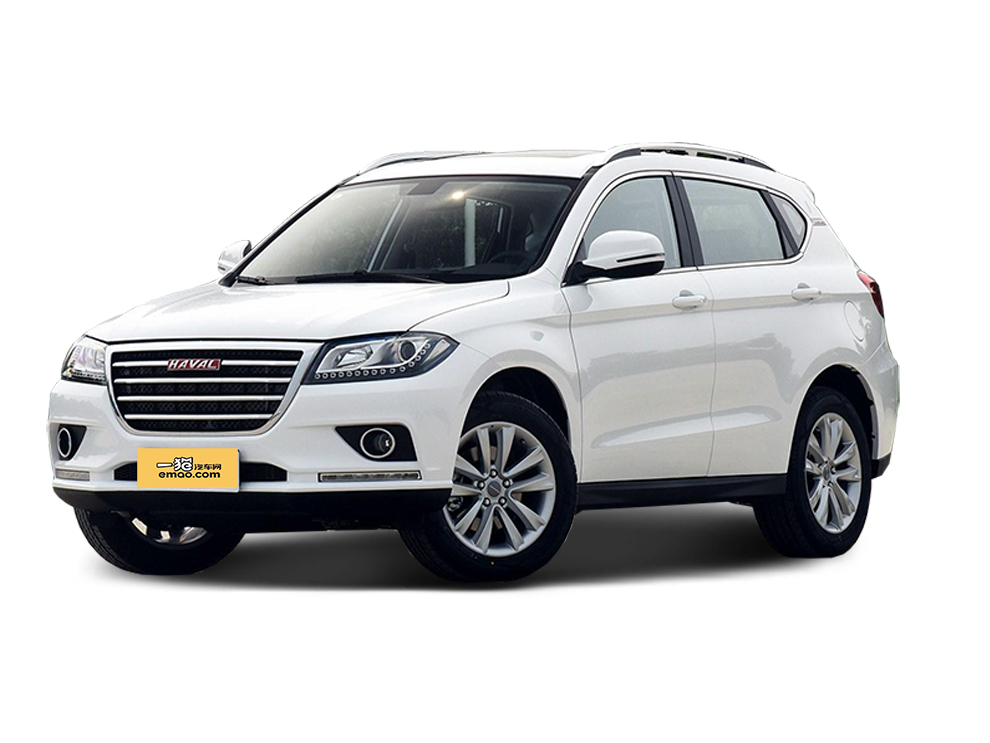##### 热度排行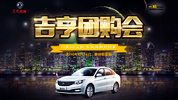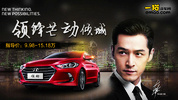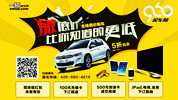• 阿斯顿·马丁
• 奥迪
• 阿尔法罗密欧
• ALPINA

• 巴博斯
• 宝骏
• 宝马
• 保时捷
• 北汽制造
• 奔驰
• 奔腾
• 本田
• 比亚迪
• 标致
• 别克
• 宾利
• 布加迪
• 北汽威旺
• 北京
• 北汽绅宝
• 北汽幻速
• 北汽新能源
• 宝沃
• 比速汽车
• 北汽道达

• 昌河
• 长安
• 长城
• 长安商用
• 成功汽车
• 长江EV
• 长安轻车型

• 大众
• 道奇
• 东风
• 东风风神
• 东风小康
• 东南
• DS
• 东风风行
• 东风风度
• 东风风光

• 法拉利
• 菲亚特
• 丰田
• 福迪
• 福特
• 福田汽车
• 福汽启腾
• 风诺

• GMC
• 广汽传祺
• 广汽吉奥
• 观致

• 哈飞
• 海格
• 海马
• 华泰
• 黄海
• 恒天
• 红旗
• 哈弗
• 华颂
• 华凯
• 华泰新能源
• 汉腾汽车

• Jeep
• 江淮
• 江铃
• 捷豹
• 金杯
• 九龙
• 吉利汽车
• 金旅
• 金龙
• 江铃集团轻汽
• 江铃集团新能源
• 君马汽车
• 捷途

• 开瑞
• 凯迪拉克
• 科尼赛克
• 克莱斯勒
• KTM
• 卡威
• 凯翼
• 康迪
• 康迪电动汽车集团

• 猎豹汽车
• 兰博基尼
• 劳斯莱斯
• 雷克萨斯
• 雷诺
• 理念
• 力帆
• 莲花汽车
• 林肯
• 铃木
• 陆风
• 路虎
• 路特斯
• 领志
• 领克
• 零跑汽车

• MG
• MINI
• 马自达
• 玛莎拉蒂
• 迈凯伦
• 摩根

• 纳智捷

• 讴歌
• 欧宝
• 欧朗
• 欧拉
• 欧尚汽车

• 帕加尼

• 奇瑞
• 启辰
• 起亚
• 前途
• 庆铃汽车

• 日产
• 荣威
• 瑞麒汽车
• 如虎
• 瑞驰

• 上汽大通
• smart
• 三菱
• 双环
• 双龙
• 斯巴鲁
• 斯柯达
• 萨博
• 思铭
• 赛麟
• SWM斯威汽车

• TESLA
• 泰卡特
• 腾势

• 威麟
• 威兹曼
• 沃尔沃
• 五菱汽车
• 五十铃
• 潍柴英致
• WEY
• 蔚来
• 威马汽车

• 现代
• 雪佛兰
• 雪铁龙
• 西雅特
• 新特汽车
• 小鹏汽车

• 野马汽车
• 一汽
• 依维柯
• 英菲尼迪
• 永源
• 驭胜

• 中华
• 中兴
• 众泰
• 知豆
• 之诺
• 正道汽车
• A
• B
• C
• D
• E
• F
• G
• H
• I
• J
• K
• L
• M
• N
• O
• P
• Q
• R
• S
• T
• U
• V
• W
• X
• Y
• Z

• 阿斯顿·马丁
• 奥迪
• 阿尔法罗密欧
• ALPINA

• 巴博斯
• 宝骏
• 宝马
• 保时捷
• 北汽制造
• 奔驰
• 奔腾
• 本田
• 比亚迪
• 标致
• 别克
• 宾利
• 布加迪
• 北汽威旺
• 北京
• 北汽绅宝
• 北汽幻速
• 北汽新能源
• 宝沃
• 比速汽车
• 北汽道达

• 昌河
• 长安
• 长城
• 长安商用
• 成功汽车
• 长江EV
• 长安轻车型

• 大众
• 道奇
• 东风
• 东风风神
• 东风小康
• 东南
• DS
• 东风风行
• 东风风度
• 东风风光

• 法拉利
• 菲亚特
• 丰田
• 福迪
• 福特
• 福田汽车
• 福汽启腾
• 风诺

• GMC
• 广汽传祺
• 广汽吉奥
• 观致

• 哈飞
• 海格
• 海马
• 华泰
• 黄海
• 恒天
• 红旗
• 哈弗
• 华颂
• 华凯
• 华泰新能源
• 汉腾汽车

• Jeep
• 江淮
• 江铃
• 捷豹
• 金杯
• 九龙
• 吉利汽车
• 金旅
• 金龙
• 江铃集团轻汽
• 江铃集团新能源
• 君马汽车
• 捷途

• 开瑞
• 凯迪拉克
• 科尼赛克
• 克莱斯勒
• KTM
• 卡威
• 凯翼
• 康迪
• 康迪电动汽车集团

• 猎豹汽车
• 兰博基尼
• 劳斯莱斯
• 雷克萨斯
• 雷诺
• 理念
• 力帆
• 莲花汽车
• 林肯
• 铃木
• 陆风
• 路虎
• 路特斯
• 领志
• 领克
• 零跑汽车

• MG
• MINI
• 马自达
• 玛莎拉蒂
• 迈凯伦
• 摩根

• 纳智捷

• 讴歌
• 欧宝
• 欧朗
• 欧拉
• 欧尚汽车

• 帕加尼

• 奇瑞
• 启辰
• 起亚
• 前途
• 庆铃汽车

• 日产
• 荣威
• 瑞麒汽车
• 如虎
• 瑞驰

• 上汽大通
• smart
• 三菱
• 双环
• 双龙
• 斯巴鲁
• 斯柯达
• 萨博
• 思铭
• 赛麟
• SWM斯威汽车

• TESLA
• 泰卡特
• 腾势

• 威麟
• 威兹曼
• 沃尔沃
• 五菱汽车
• 五十铃
• 潍柴英致
• WEY
• 蔚来
• 威马汽车

• 现代
• 雪佛兰
• 雪铁龙
• 西雅特
• 新特汽车
• 小鹏汽车

• 野马汽车
• 一汽
• 依维柯
• 英菲尼迪
• 永源
• 驭胜

• 中华
• 中兴
• 众泰
• 知豆
• 之诺
• 正道汽车
• A
• B
• C
• D
• E
• F
• G
• H
• I
• J
• K
• L
• M
• N
• O
• P
• Q
• R
• S
• T
• U
• V
• W
• X
• Y
• Z

• 阿斯顿·马丁
• 奥迪
• 阿尔法罗密欧
• ALPINA

• 巴博斯
• 宝骏
• 宝马
• 保时捷
• 北汽制造
• 奔驰
• 奔腾
• 本田
• 比亚迪
• 标致
• 别克
• 宾利
• 布加迪
• 北汽威旺
• 北京
• 北汽绅宝
• 北汽幻速
• 北汽新能源
• 宝沃
• 比速汽车
• 北汽道达

• 昌河
• 长安
• 长城
• 长安商用
• 成功汽车
• 长江EV
• 长安轻车型

• 大众
• 道奇
• 东风
• 东风风神
• 东风小康
• 东南
• DS
• 东风风行
• 东风风度
• 东风风光

• 法拉利
• 菲亚特
• 丰田
• 福迪
• 福特
• 福田汽车
• 福汽启腾
• 风诺

• GMC
• 广汽传祺
• 广汽吉奥
• 观致

• 哈飞
• 海格
• 海马
• 华泰
• 黄海
• 恒天
• 红旗
• 哈弗
• 华颂
• 华凯
• 华泰新能源
• 汉腾汽车

• Jeep
• 江淮
• 江铃
• 捷豹
• 金杯
• 九龙
• 吉利汽车
• 金旅
• 金龙
• 江铃集团轻汽
• 江铃集团新能源
• 君马汽车
• 捷途

• 开瑞
• 凯迪拉克
• 科尼赛克
• 克莱斯勒
• KTM
• 卡威
• 凯翼
• 康迪
• 康迪电动汽车集团

• 猎豹汽车
• 兰博基尼
• 劳斯莱斯
• 雷克萨斯
• 雷诺
• 理念
• 力帆
• 莲花汽车
• 林肯
• 铃木
• 陆风
• 路虎
• 路特斯
• 领志
• 领克
• 零跑汽车

• MG
• MINI
• 马自达
• 玛莎拉蒂
• 迈凯伦
• 摩根

• 纳智捷

• 讴歌
• 欧宝
• 欧朗
• 欧拉
• 欧尚汽车

• 帕加尼

• 奇瑞
• 启辰
• 起亚
• 前途
• 庆铃汽车

• 日产
• 荣威
• 瑞麒汽车
• 如虎
• 瑞驰

• 上汽大通
• smart
• 三菱
• 双环
• 双龙
• 斯巴鲁
• 斯柯达
• 萨博
• 思铭
• 赛麟
• SWM斯威汽车

• TESLA
• 泰卡特
• 腾势

• 威麟
• 威兹曼
• 沃尔沃
• 五菱汽车
• 五十铃
• 潍柴英致
• WEY
• 蔚来
• 威马汽车

• 现代
• 雪佛兰
• 雪铁龙
• 西雅特
• 新特汽车
• 小鹏汽车

• 野马汽车
• 一汽
• 依维柯
• 英菲尼迪
• 永源
• 驭胜

• 中华
• 中兴
• 众泰
• 知豆
• 之诺
• 正道汽车
• A
• B
• C
• D
• E
• F
• G
• H
• I
• J
• K
• L
• M
• N
• O
• P
• Q
• R
• S
• T
• U
• V
• W
• X
• Y
• Z